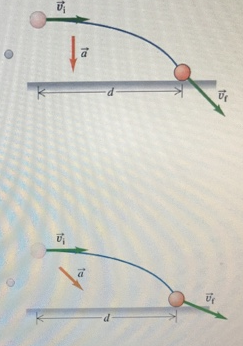# Problem: A ball thrown horizontally with speed vi 20.0 m/s travels a horizontal distance of 55.0 m before hitting the ground. From what height h was the ball thrown? Which diagram represents an accurate visual overview of the ball?

###### FREE Expert Solution

Uniform accelerated motion (UAM) equations:

We'll solve for the flight time from the horizontal motion.

vx = vi = 20 m/s

d = vi•t

95% (148 ratings)###### Problem Details

A ball thrown horizontally with speed vi 20.0 m/s travels a horizontal distance of 55.0 m before hitting the ground. From what height h was the ball thrown?

Which diagram represents an accurate visual overview of the ball?